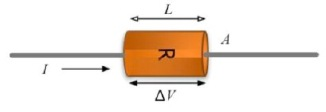Teaching Physics with the Physics Suite Edward F. Redish Problems Sorted by Type | Problems Sorted by Subject | Problems Sorted by Chapter in UP

Mechanism in a resistor

 A steady current is flowing through a resistor that is made out of an electrically uniform substance. It is well described by Ohm’s law. The resistor has the dimensions and electrical measurements as indicated in the figure. For each of the following, choose all of the statements that are correct. If none work, put N.A. Which of the following statements are likely to be at least partially responsible for the validity of Ohm’s law for this resistor?

1. The resistoris made from a material that is a conductor.
2. The resistor is made froma material that is an insulator.
3. Moving charges in the resistor feel a resisting frictional force that is independent of their velocity.
4. Moving charges in the resistor feel a resisting drag force that is linearly proportional to their velocity.
5. None of the above statements canB. be relevant to the validity of Ohm’s law.

B. Suppose that the original resistor is replaced by a resistor of the same length and identical material but twice the cross sectional area. Further suppose that the potential drop across the resistor is the same as for the original. The current through the new resistor will be

1. The same as for the original resistor.
2. Twice as big as for the original resistor.
3. Half as big as for the original resistor.
4. Some other multiple of the original current.
5. You can’t tell from the information given.

C. Suppose that the original resistor is replaced by a resistor of the same cross sectional area and identical material but twice the length. Further suppose that the potential drop across the resistor is the same as for the original. The current through the new resistor will be

1. The same as for the original resistor.
2. Twice as big as for the original resistor.
3. Half as big as for the original resistor.
4. Some other multiple of the original current.
5. You can’t tell from the information given.

D. Suppose that the original resistor is replaced by a resistor of the same physical dimensions but made of a material that has half the density of charges to carry current, but each carrier has double the charge of those in the original resistor. Further suppose that the potential drop across the resistor is the same as for the original The current through the new resistor will be

1. The same as for the original resistor.
2. Twice as big as for the original resistor.
3. Half as big as for the original resistor.
4. Some other multiple of the original current.
5. You can’t tell from the information given.